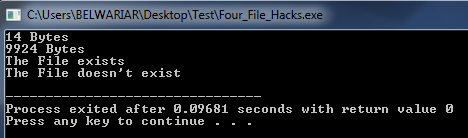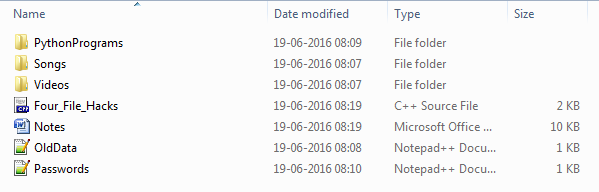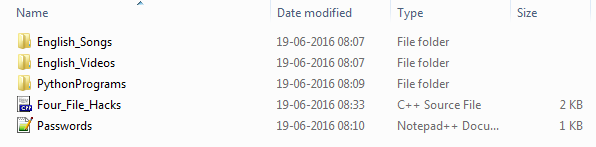# Four File Handling Hacks which every C/C++ Programmer should know

• Difficulty Level : Medium
• Last Updated : 13 Jun, 2022

We will discuss about four file hacks listed as below-

1. Rename – Rename a file using C/C++
2. Remove – Remove a file using C/C++
3. File Size – Get the file size using C/C++
4. Check existence – Check whether a file exists or not in C/C++

## C++

 `// C++ Program to demonstrate the``// four file hacks every C/C++ must know` `// Note that we are assuming that the files``// are present in the same file as the program``// before doing the below four hacks` `#include ``#include ``using` `namespace` `std;` `// A Function to get the file size``unsigned ``long` `long` `int` `fileSize(``const` `char` `*filename)``{``    ``// Open the file``    ``FILE` `*fh = ``fopen``(filename, ``"rb"``);``    ``fseek``(fh, 0, SEEK_END);``    ``unsigned ``long` `long` `int` `size = ``ftell``(fh);``    ``fclose``(fh);` `    ``return` `size;``}` `// A Function to check if the file exists or not``bool` `fileExists(``const` `char` `* fname)``{``    ``FILE` `*file;``    ``if` `(file = ``fopen``(fname, ``"r"``))``    ``{``        ``fclose``(file);``        ``return` `true``;``    ``}``    ``return` `false``;``}` `// Driver Program to test above functions``int` `main()``{``    ``cout << fileSize(``"Passwords.txt"``) << ``" Bytes\n"``;``    ``cout << fileSize(``"Notes.docx"``) << ``" Bytes\n"``;` `    ``if` `(fileExists(``"OldData.txt"``) == ``true` `)``        ``cout << ``"The File exists\n"``;``    ``else``        ``cout << ``"The File doen't exist\n"``;` `    ``rename``(``"Videos"``, ``"English_Videos"``);``    ``rename``(``"Songs"``, ``"English_Songs"``);` `    ``remove``(``"OldData.txt"``);``    ``remove``(``"Notes.docx"``);` `    ``if` `(fileExists(``"OldData.txt"``) == ``true` `)``        ``cout << ``"The File exists\n"``;``    ``else``        ``cout << ``"The File doesn't exist\n"``;` `    ``return` `0;``}` `// This code is contributed by sarajadhav12052009`

## C

 `// A C Program to demonstrate the``// four file hacks every C/C++ must know` `// Note that we are assuming that the files``// are present in the same file as the program``// before doing the below four hacks``#include``#include``#include` `// A Function to get the file size``unsigned ``long` `long` `int` `fileSize(``const` `char` `*filename)``{``    ``// Open the file``    ``FILE` `*fh = ``fopen``(filename, "rb");``    ``fseek``(fh, 0, SEEK_END);``    ``unsigned ``long` `long` `int` `size = ``ftell``(fh);``    ``fclose``(fh);` `    ``return` `(size);``}` `// A Function to check if the file exists or not``bool` `fileExists(``const` `char` `* fname)``{``    ``FILE` `*file;``    ``if` `(file = ``fopen``(fname, "r"))``    ``{``        ``fclose``(file);``        ``return` `(``true``);``    ``}``    ``return` `(``false``);``}` `// Driver Program to test above functions``int` `main()``{``    ``printf``("%llu Bytes\n", fileSize("Passwords.txt"));``    ``printf``("%llu Bytes\n", fileSize("Notes.docx"));` `    ``if` `(fileExists("OldData.txt") == ``true` `)``        ``printf``("The File exists\n");``    ``else``        ``printf``("The File doen't exist\n");` `    ``rename``("Videos", "English_Videos");``    ``rename``("Songs", "English_Songs");` `    ``remove``("OldData.txt");``    ``remove``("Notes.docx");` `    ``if` `(fileExists("OldData.txt") == ``true` `)``        ``printf``("The File exists\n");``    ``else``        ``printf``("The File doesn't exist\n");` `    ``return` `0;``}`

Output:Screenshot before executing the program :Screenshot after executing the program :This article is contributed by Rachit Belwariar. If you like GeeksforGeeks and would like to contribute, you can also write an article and mail your article to review-team@geeksforgeeks.org. See your article appearing on the GeeksforGeeks main page and help other Geeks. Please write comments if you find anything incorrect, or you want to share more information about the topic discussed above

My Personal Notes arrow_drop_up